# imtransform

Apply 2-D spatial transformation to image

`imtransform` is not recommended. Use `imwarp` instead.

## Syntax

``B = imtransform(A,tform)``
``B = imtransform(A,tform,interp)``
``B = imtransform(___,Name,Value)``
``[B,xdata,ydata] = imtransform(___)``

## Description

example

````B = imtransform(A,tform)` transforms image `A` according to the 2-D spatial transformation defined by `tform`, and returns the transformed image, `B`.If `A` is a color image, then `imtransform` applies the same 2-D transformation to each color channel. Likewise, if `A` is a volume or image sequence with three or more dimensions, then `imtransform` applies the same 2-D transformation to all 2-D planes along the higher dimensions. For arbitrary-dimensional array transformations, use `tformarray`.```
````B = imtransform(A,tform,interp)` specifies the form of interpolation to use.```
````B = imtransform(___,Name,Value)` uses name-value pairs to control various aspects of the spatial transformation.```

example

````[B,xdata,ydata] = imtransform(___)` also returns the extent of the output image `B` in the output X-Y space. By default, `imtransform` calculates `xdata` and `ydata` automatically so that `B` contains the entire transformed image `A`. However, you can override this automatic calculation by specifying values for the `XData` and `YData` name-value pair input arguments.```

## Examples

### Simple Transformation

Apply a horizontal shear to a grayscale image.

```I = imread('cameraman.tif'); tform = maketform('affine',[1 0 0; .5 1 0; 0 0 1]); J = imtransform(I,tform); imshow(J)```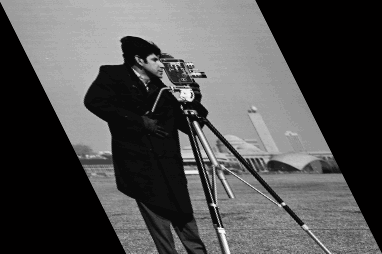### Projective Transformation

Map a square to a quadrilateral with a projective transformation. Set up an input coordinate system so that the input image fills the unit square with vertices (0 0), (1 0), (1 1), (0 1).

```I = imread('cameraman.tif'); udata = [0 1]; vdata = [0 1];```

Transform to a quadrilateral with vertices (-4 2), (-8 3), (-3 -5), (6 3).

```tform = maketform('projective',[ 0 0; 1 0; 1 1; 0 1],... [-4 2; -8 -3; -3 -5; 6 3]);```

Fill with gray and use bicubic interpolation. Make the output size the same as the input size.

```[B,xdata,ydata] = imtransform(I,tform,'bicubic', ... 'udata',udata,... 'vdata',vdata,... 'size',size(I),... 'fill',128); subplot(1,2,1); imshow(I,'XData',udata,'YData',vdata) subplot(1,2,2); imshow(B,'XData',xdata,'YData',ydata)```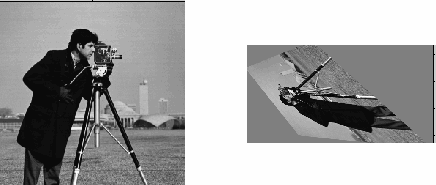### Image Registration

Read an aerial photo into the MATLAB® workspace and view it.

```unregistered = imread('westconcordaerial.png'); figure imshow(unregistered)```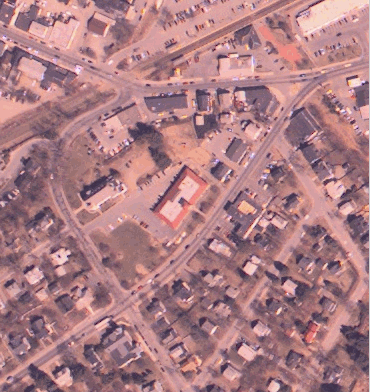Read an orthophoto into the MATLAB workspace and view it.

```figure imshow('westconcordorthophoto.png')```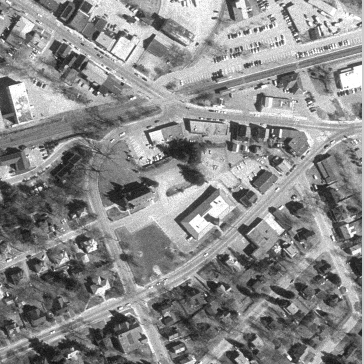Load control points that were previously picked.

`load westconcordpoints`

Create a transformation structure for a projective transformation using the points.

`t_concord = cp2tform(movingPoints,fixedPoints,'projective');`

Get the width and height of the orthophoto, perform the transformation, and view the result.

```info = imfinfo('westconcordorthophoto.png'); registered = imtransform(unregistered,t_concord,... 'XData',[1 info.Width],'YData',[1 info.Height]); figure imshow(registered)```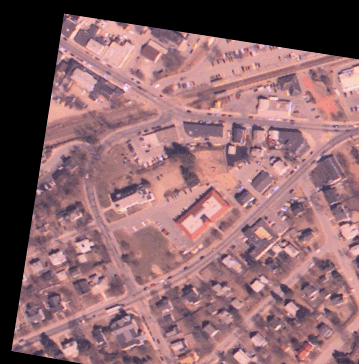## Input Arguments

collapse all

Image to be transformed, specified as a numeric or logical array of any dimension.

Data Types: `single` | `double` | `int8` | `int16` | `int32` | `int64` | `uint8` | `uint16` | `uint32` | `uint64` | `logical`

Transformation structure, specified as a struct, such as returned by `maketform` or `cp2tform`. The first dimension of the transformation is the horizontal or x-coordinate, and the second dimension is the vertical or y-coordinate. This convention is the reverse of the array subscripting convention in MATLAB.

Interpolation method, specified as one of these values.

Interpolation MethodDescription
`'bilinear'`Linear interpolation
`'nearest'`Nearest-neighbor interpolation—the output pixel is assigned the value of the pixel that the point falls within. No other pixels are considered.
`'bicubic'`Cubic interpolation
`resampler` structure`resampler` structure returned by `makeresampler`. This option allows more control over how `imtransform` performs resampling.

Data Types: `char`

### Name-Value Pair Arguments

Specify optional comma-separated pairs of `Name,Value` arguments. `Name` is the argument name and `Value` is the corresponding value. `Name` must appear inside quotes. You can specify several name and value pair arguments in any order as `Name1,Value1,...,NameN,ValueN`.

Example: `B = imtransform(A,T,'FillValues',128)`

Spatial extent of input image `A` in the U-V input space, specified as 2-element numeric vectors. The values of `UData` and `VData` represent coordinates in the world coordinate system. The two elements of `UData` give the u-coordinates (horizontal) of the first and last columns of `A`, respectively. The two elements of `VData` give the v-coordinates (vertical) of the first and last rows of `A`.

By default, the spatial extent of `A` in the U-V space is the same as the image extent in intrinsic coordinates. In other words, the default value of `UData` is `[1 size(A,2)]` and the default value of `VData` is ```[1 size(A,1)]```.

Spatial extent of transformed image `B` in the X-Y input space, specified as 2-element numeric vectors. The values of `XData` and `YData` represent coordinates in the world coordinate system. The two elements of `XData` give the x-coordinates (horizontal) of the first and last columns of `B`, respectively. The two elements of `YData` give the y-coordinates (vertical) of the first and last rows of `B`.

If you do not specify `XData` and `YData`, then `imtransform` estimates values that contain the entire transformed output image. To determine these values, `imtransform` uses the `findbounds` function.

Size of pixels in X-Y output space, specified as a numeric scalar or 2-element numeric vector. If `XYScale` is a scalar, then output pixels are square and `XYScale` specifies the side length. Otherwise, the two elements of `XYScale` specify the width and height of each output pixel in X-Y space, respectively.

The default value of `XYScale` depends on whether you specify `Size`:

• If you specify `Size`, then `imtransform` calculates `XYScale` from `Size`, `XData`, and `YData`.

• If you do not specify `Size`, then `imtransform` uses the scale of the input pixels for `XYScale`, except in cases where an excessively large output image would result.

### Note

In cases where preserving the scale of the input image would result in an excessively large output image, the `imtransform` function automatically increases the value of `XYScale`. To ensure that the output pixel scale matches the input pixel scale, specify the `XYScale` parameter. For example, call `imtransform` as shown in the following syntax:

`B = imtransform(A,T,'XYScale',1)`

Size of transformed image `B`, specified as a 2-element vector of positive integers. The two elements of `Size` specify the number of rows and columns of the output image `B`, respectively. For higher dimensions, `imtransform` takes the size of `B` directly from the size of input image `A`. Thus, `size(B,k)` equals `size(A,k)` for `k > 2`.

If you do not specify `Size`, then `imtransform` derives this value from `XData`, `YData`, and `XYScale`.

Fill value used for output pixels outside the input image boundaries, specified as the comma-separated pair consisting of `'FillValues'` and a numeric scalar or numeric array. Fill values are used for output pixels when the corresponding inverse transformed location in the input image is completely outside the input image boundaries.

• If the input image `A` is 2-D, then `FillValues` must be a scalar.

• If `A` is 3-D or N-D, then `FillValues` can be an array whose size satisfies the following constraint: `size(FillValues,k)` must equal either `size(A,k+2)` or `1`.

For example, if `A` is a `uint8` RGB image that is 200-by-200-by-3, then possibilities for `'FillValues'` include the following values.

ValueFill
`0`Fill with black
`[0;0;0]`Fill with black
`255`Fill with white
`[255;255;255]`Fill with white
`[0;0;255]`Fill with blue
`[255;255;0]`Fill with yellow

For a second example, if `A` is 4-D with size 200-by-200-by-3-by-10, then you can specify `'FillValues'` as a scalar, 1-by-10 vector, 3-by-1 vector, or 3-by-10 matrix.

## Output Arguments

collapse all

Transformed image, returned as a numeric or logical array of the same dimensionality as the input image `A`.

Horizontal extent of the transformed image `B` in X-Y output space, returned as a 2-element numeric vector. The two elements of `xdata` give the x-coordinates (horizontal) of the first and last columns of `B` in the world coordinate system, respectively.

### Note

The first element of `xdata` always equals the first element of the `XData` argument, if specified. However, sometimes the second element of `xdata` does not exactly equal the second element of `XData`. The values differ either because of the need for an integer number of rows and columns, or because you specified values for `XData`, `YData`, `XYScale`, and `Size` that are not entirely consistent.

Vertical extent of the transformed image `B` in X-Y output space, returned as a 2-element numeric vector. The two elements of `ydata` give the y-coordinates (vertical) of the first and last rows of `B` in the world coordinate system, respectively.

### Note

The first element of `ydata` always equals the first element of the `YData` argument, if specified. However, sometimes the second element of `ydata` does not exactly equal the second element of `YData`. The values differ either because of the need for an integer number of rows and columns, or because you specified values for `XData`, `YData`, `XYScale`, and `Size` that are not entirely consistent.

## Tips

• Image Registration. The `imtransform` function automatically shifts the origin of your output image to make as much of the transformed image visible as possible. If you use `imtransform` to do image registration, the syntax ```B = imtransform(A,tform)``` can produce unexpected results. To control the spatial location of the output image, set `XData` and `YData` explicitly.

• Pure Translation. Calling the `imtransform` function with a purely translational transformation results in an output image that is exactly like the input image unless you specify `XData` and `YData` values in your call to `imtransform`. For example, if you want the output to be the same size as the input revealing the translation relative to the input image, call `imtransform` as shown in the following syntax:

```B = imtransform(A,T,'XData',[1 size(A,2)],... 'YData',[1 size(A,1)])```

• Transformation Speed. If you do not specify the output-space location for `B` using `XData` and `YData`, then `imtransform` estimates the location automatically using the function `findbounds`. You can use `findbounds` as a quick forward-mapping option for some commonly used transformations, such as affine or projective. For transformations that do not have a forward mapping, such as polynomial transformations computed by `fitgeotrans`, `findbounds` can take much longer. If you can specify `XData` and `YData` directly for such transformations, then `imtransform` may run noticeably faster.

• Clipping. The automatic estimate of `XData` and `YData` using `findbounds` sometimes clips the output image. To avoid clipping, set `XData` and `YData` directly.

• Arbitrary Dimensional Transformations. Use a 2-D transformation for `tform` when using `imtransform`. For arbitrary-dimensional array transformations, see `tformarray`.